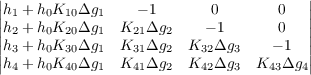#### Vol. 64, No. 2, 1976

 Download this articleFor screen For printingRecent Issues Vol. 325: 1 Vol. 324: 1  2 Vol. 323: 1  2 Vol. 322: 1  2 Vol. 321: 1  2 Vol. 320: 1  2 Vol. 319: 1  2 Vol. 318: 1  2Online Archive Volume: Issue:The Journal Subscriptions Editorial Board Officers Contacts Submission Guidelines Submission Form Policies for Authors ISSN: 1945-5844 (e-only) ISSN: 0030-8730 (print) Special Issues Author Index To Appear Other MSP Journals
The solution of a Stieltjes-Volterra integral equation for rings

### Burrell Washington Helton

Vol. 64 (1976), No. 2, 419–436
##### Abstract

For a triple (h,K,g) of functions and an interval [a,x], the author defines a subdivision-refinement-type limit V (a,x;h,K,dg) of the set {V (D,h,K,Δg)} of determinants, where each subdivision D = {xi}0n of [a,x] defines an n×n determinant of the set and each determinant has the formThe following theorem is proved. If f, g, h and K are functions to a ring and g has bounded variation on [a,b], then (f,K,g) OA and f(x) = h(x) + (L) axf(t)K(x,t)dg(t) on [a,b] iff (h,K,g) OM and f(x) = V (a,x;h,K,dg) on [a,b]. The OA and OM sets are defined and sufficient conditions are proved for (f,K,g) OA and (h,K,g) OM, and for the existence of the limit V (a,x;h,K,dg), and for V (a,x;h,K,dg) = h(x) (L) axh(t)dV (t,x;1,K,dg).

Primary: 45D05
##### Milestones